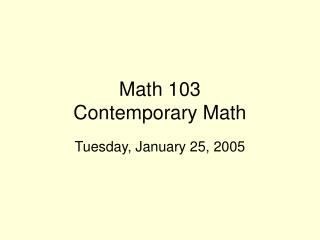DownloadDownload PresentationMath 103 Contemporary Math

# Math 103 Contemporary Math

Télécharger la présentation## Math 103 Contemporary Math

- - - - - - - - - - - - - - - - - - - - - - - - - - - E N D - - - - - - - - - - - - - - - - - - - - - - - - - - -
##### Presentation Transcript

1. Math 103 Contemporary Math Tuesday, January 25, 2005

2. The Sphere,The Torus & Flatland. • How can one distinguish the sphere from a plane (Flatland) based solely on experiences on the surface? • How can one distinguish the sphere from a torus based solely on experiences on the surface? • Use shadows?: look at shadows at the same time of day? This is a "local"  feature of the surface. • Observe "curvature"? This is also a local property. • Circumnavigate (Global)?:  Go West -> return from the East, then  go North-> return from the south. • On a sphere: • On a torus: • On a plane: • Other issues: What about strange gravity? Finding an edge? How do you know when you start?

3. Measurement and the Pythagorean Theorem (PT) • Do Pythagorean Activity Sheet • Virtual Manipulative for PT. • Discuss Pythagorean Theorem and proofs. • Over 30 proofs of the Pythagorean theorem! • Many Java Applets that visualize proofs of the Pythagorean Theorem a2 + b2 = c2

4. Outline of Video on PT[Put on reserve in library!] • Background: Similar triangles • Area of triangles = 1/2 bh • Area of parallelogram = bh • Scaling: a linear scale change of r gives area change of factor r 2. • 3 questions: running, moat, wind power... • Proof of the PT: Similar right triangles: c= a2 /c + b2 /c • applications and other proofs. • Prop. 47 of Euclid. • Dissection Proof. • Prop 31 Book  VI  Similar shapes. • Simple proof of PT using similar triangles of the triangle. • Use in 3 dimensional space.

5. Puzzles and Polygons Measuring angles, lengths and areas. • Squares, rectangles  : 90 degree/ right angle • triangles : add to 180 degrees- straight angle [Illustrated physically and with wingeometry] • parallelograms: opposite angles are congruent, sum of consecutive angles =180 degrees • Dissections, cut and paste methods of measurement. • Cutting and reassembling polygons. • The "Square Me" Puzzle

6. The triangle, quadrilateral, pentagon, and hexagon. • More on measurements of angles and areas of polygons. • A quadrilateral can be made from two triangles...so the sum of its interior angles is 2 * 180 = 360. • A pentagon can be made from 3 triangles... so the sum of its interior angles is 3* 180 = ___. If the hexagon has all angles congruent( of equal measurement) then each angle will be ___/5 = ___ degrees! • A hexagon can be made from 4 triangles... so the sum of its interior angles is 4* 180 = ___. If the hexagon has all angles congruent( of equal measurement) then each angle will be ___/6 = ___ degrees!

7. Measuring angles in Polygons

8. Tangrams • Tangrams. • Virtual Tangram Puzzle • More • Tangram ActivityUse templates to cut out pieces from larger (blue) sheets? [#Partners=3.] • Cutting and reassembling polygons.